# Standard Deviation Calculator

You can use this Standard Deviation Calculator to calculate the standard deviation, variance, mean, and the coefficient of variance for a given set of numbers.

Please provide numbers separated by comma (e.g: 7,1,8,5), space (e.g: 7 1 8 5) or line break and press the "Calculate" button.

Standard Deviation (SD) Calculator

Results

## Standard Deviation

Standard deviation is used when it comes to statistics and probability theory. It is used to measure both variability and diversity and shows the precision of your data.

When you calculate standard deviation, you are calculating the square root of its variance. A low standard deviation will indicate that the entered data points are most likely to be closer to the mean, compared to if there is a high standard deviation which is an indication that the entered data points are most likely further away from the mean.

Calculating both the variance and the standard deviation depends on not only the set of numbers you are using to calculate them but whether the set of numbers is a population or a sample.

## Variance and Standard Deviation of a Population

When calculating the standard deviation of a population you are calculating the variation of data within a population. The standard deviation is usually an unknown constant.

• Our Standard Deviation Calculator is appropriate for carrying out any mathematical calculations that consist of the formulae and algorithms found below.

Standard deviation (σ) and Variance (σ2) of the population are given as: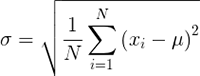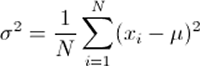• σ = Standard Deviation of the Population
• σ2 = Variance of the Population
• x1, ..., xN = the Population Data Set
• μ = Mean of the Population Data Set
• N = Size of the Population Data Set

## Variance and Standard Deviation of a Sample

When calculating the standard deviation of a sample, you are calculating an estimate of the standard deviation of a population. Variance (s2) and the Standard Deviation (s) of the sample are calculated using the following formulae.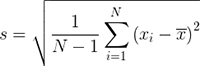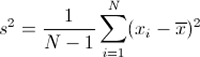• s = Standard Deviation of the Sample
• s2 = Variance of the Sample
• x1, ..., xN = the Sample Data Set
• x̄ = Mean of the Sample Data Set
• N = Size of the Sample Data Set

## Coefficient of Variation (CV)

When standard deviation is expressed as a percentage of the mean value, it is known as the Coefficient of Variation.

To calculate the Coefficient of Variation, use the following formula:

• Coefficient of Variation (CV) = Standard Deviation (σ) / Mean Value (μ)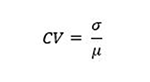To calculate the mean, use the following formula: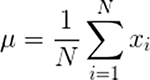The formula found above will depict the Coefficient of Variation's meaning which can then be applied to not only the population but the sample of a certain distribution. You can also express the coefficient of variation as a percentage by multiplying your result from the above formula by 100. This is a useful way to measure variability when multiple groups are given and are in different measurement units.

## Standard Deviation Calculation Examples

Consider the set X of numbers 1, 2, 3, 4, 5

Step 1 :

• Mean = Sum of X values / N (Number of Values)
• = (1 + 2 + 3 + 4 + 5) / 5
• = 15 / 5
• = 3

Step 2 :

• In order to find the variance,
• Subtract the mean from each of the values,
• 1 - 3 = -2
• 2 - 3 = -1
• 3 - 3 = 0
• 4 - 3 = 1
• 5 - 3 = 2
• Now square all of the answers that you had gotten from subtraction.
• (-2)² = 4
• (-1)² = 1
• (0)² = 0
• (1)² = 1
• (2)² = 4
• Add all of the squared numbers,
• 4 + 1 + 0 + 1 + 4 = 10
• Divide the sum of squares by (n-1)
• 10 / (5 - 1) = 10 / 4 = 2.5
• Therefore, Variance = 2.5

Step 3 :

• To find the standard deviation, find the square root of variance,
• √2.5 = 1.581
• Therefore, standard deviation is 1.581
• To find minimum and maximum standard deviation,
• Minimum SD = Mean − SD
• = 3 - 1.581
• = 1.419
• Maximum SD = Mean + SD
• =3 + 1.581
• = 4.581

Step 4 :

• To find the population standard deviation,
• Divide the sum of squares found in step 2 by n
• 10 / 5 = 2
• Find the square root of 2, √2 = 1.414

You may also be interested in our Standard Error Calculator or Six Sigma Calculator